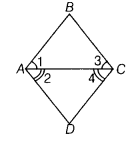# In the given' figure,∠1 =∠3 and ∠2 =∠4. Using Euclid’s axiom, show that ∠A =∠C

In the given’ figure,∠1 =∠3 and∠2 =∠4. Using Euclid’s axiom, show that ∠A =∠C.Given, ∠1 = ∠3,…(i)
and ∠2 =∠4 …(ii)
Euclid’s second axiom says that, if equals are added to equals, then the wholes are equal.

On adding Eqs. (i) and (ii), we get
∠1 +∠2 =∠3 + ∠4
=> ∠4 =∠C